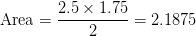# Basic Geometry : How to find the area of a right triangle

## Example Questions

### Example Question #41 : How To Find The Area Of A Right Triangle

Find the area of a right triangle with leg lengths ofand.Explanation:

Recall how to find the area of a right triangle: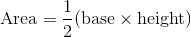Because the legs of the right triangle form a right angle, the legs are also the base and the height.To find the area of the right triangle, just plug in the values for the lengths of the legs into the equation given above.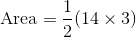Simplify.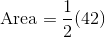Solve.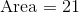### Example Question #42 : How To Find The Area Of A Right Triangle

Find the area of a right triangle with leg lengths ofand.Explanation:

Recall how to find the area of a right triangle:Because the legs of the right triangle form a right angle, the legs are also the base and the height.To find the area of the right triangle, just plug in the values for the lengths of the legs into the equation given above.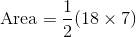Simplify.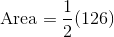Solve.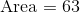### Example Question #43 : How To Find The Area Of A Right Triangle

Find the area of a right triangle with leg lengths ofand.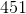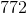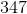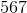Explanation:

Recall how to find the area of a right triangle:Because the legs of the right triangle form a right angle, the legs are also the base and the height.To find the area of the right triangle, just plug in the values for the lengths of the legs into the equation given above.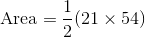Simplify.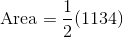Solve.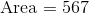### Example Question #44 : How To Find The Area Of A Right Triangle

Find the area of a right triangle with leg lengths ofand.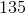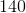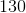Explanation:

Recall how to find the area of a right triangle:Because the legs of the right triangle form a right angle, the legs are also the base and the height.To find the area of the right triangle, just plug in the values for the lengths of the legs into the equation given above.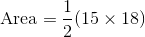Simplify.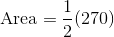Solve.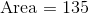### Example Question #45 : How To Find The Area Of A Right Triangle

Find the area.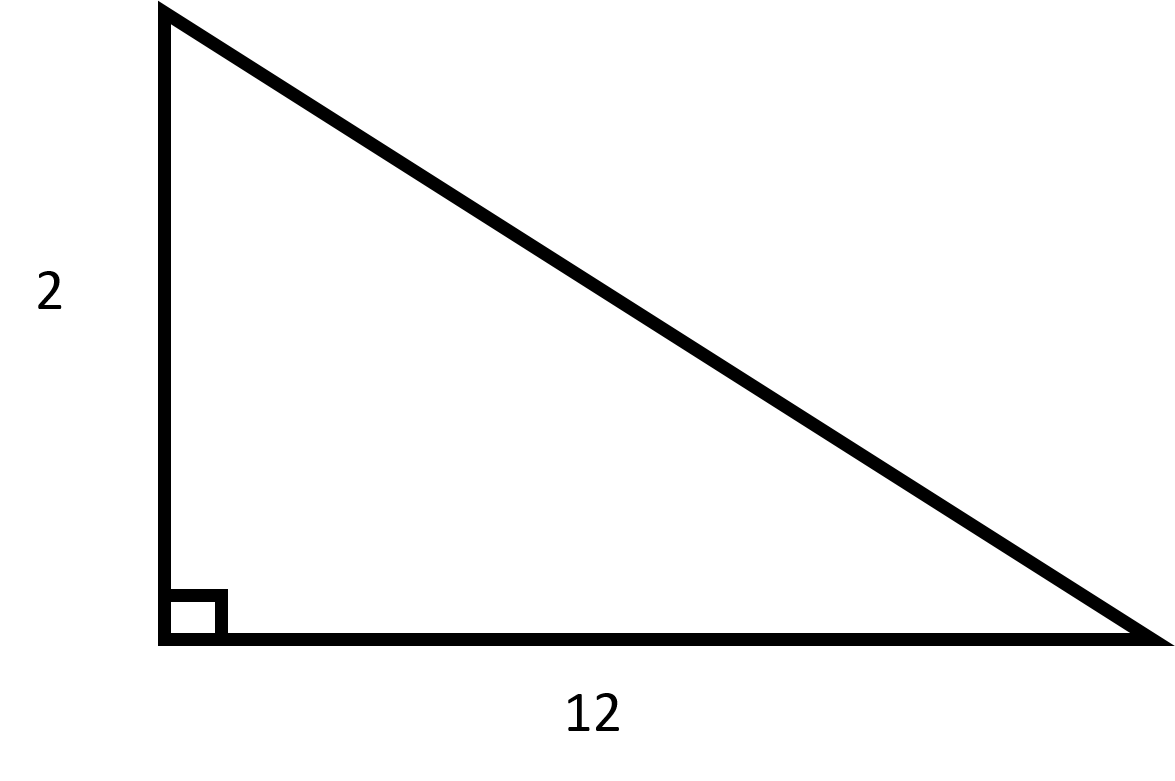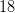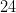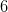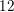Explanation:

Recall how to find the area of a triangle: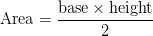Since this is a right triangle, the base and the height are the two leg lengths given.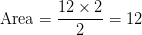### Example Question #46 : How To Find The Area Of A Right Triangle

Find the area.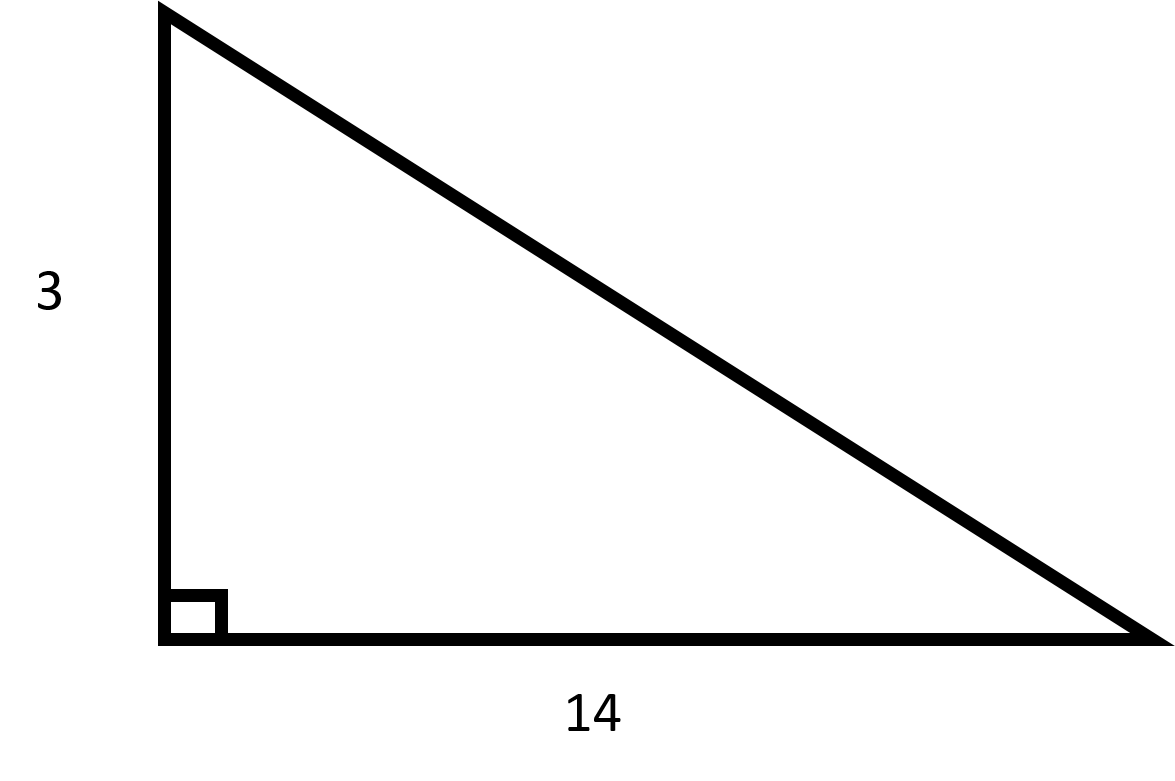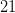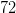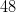Explanation:

Recall how to find the area of a triangle:Since this is a right triangle, the base and the height are the two leg lengths given.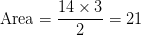### Example Question #47 : How To Find The Area Of A Right Triangle

Find the area.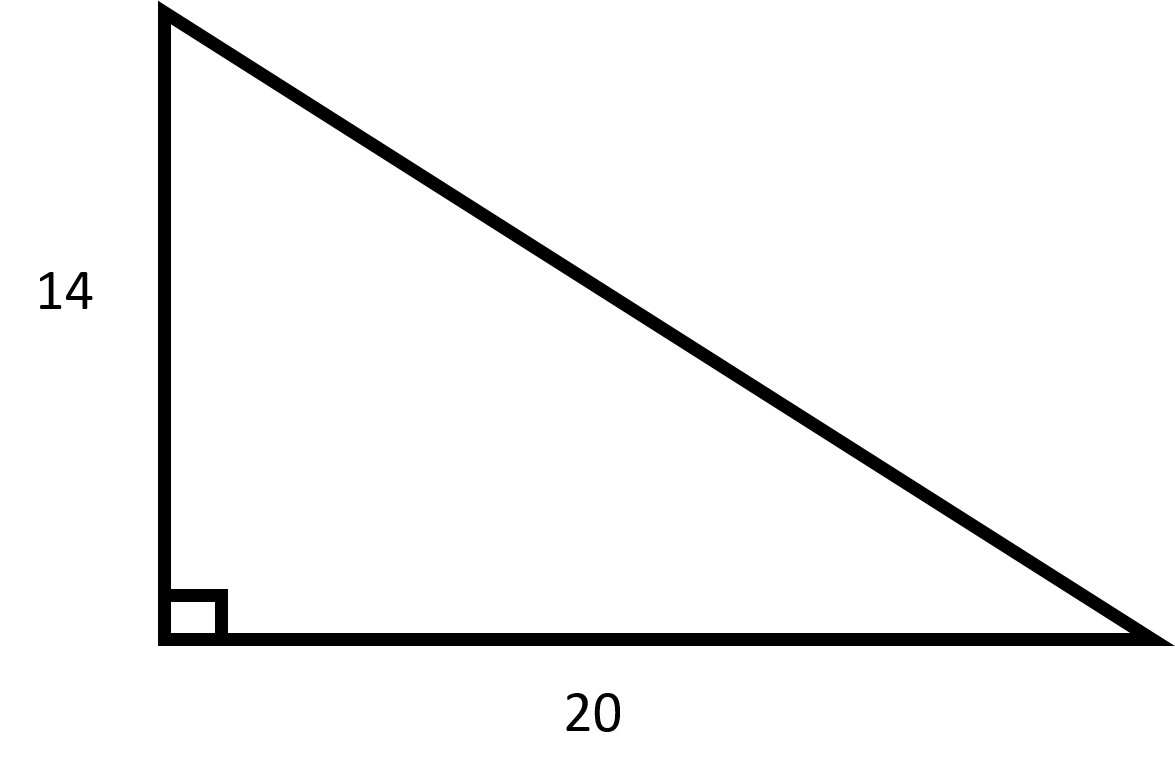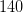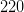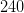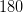Explanation:

Recall how to find the area of a triangle:Since this is a right triangle, the base and the height are the two leg lengths given.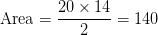### Example Question #48 : How To Find The Area Of A Right Triangle

Find the area.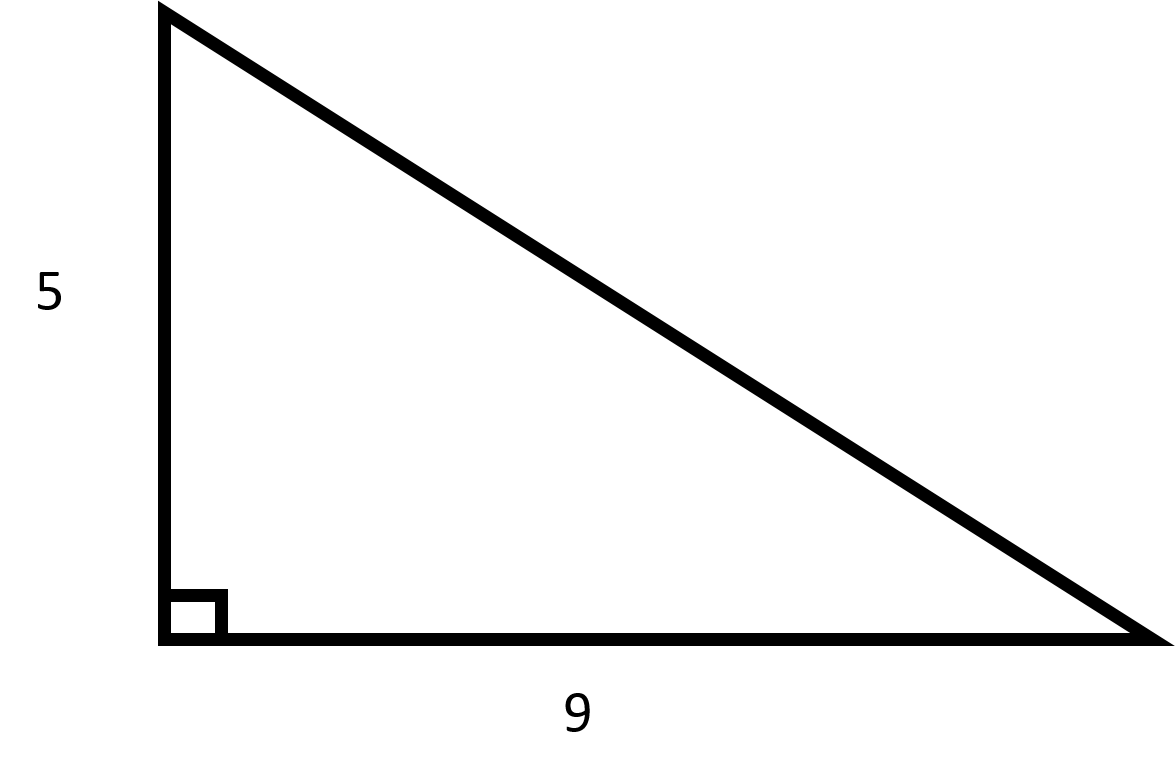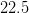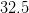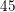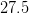Explanation:

Recall how to find the area of a triangle:Since this is a right triangle, the base and the height are the two leg lengths given.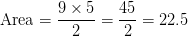### Example Question #49 : How To Find The Area Of A Right Triangle

Find the area.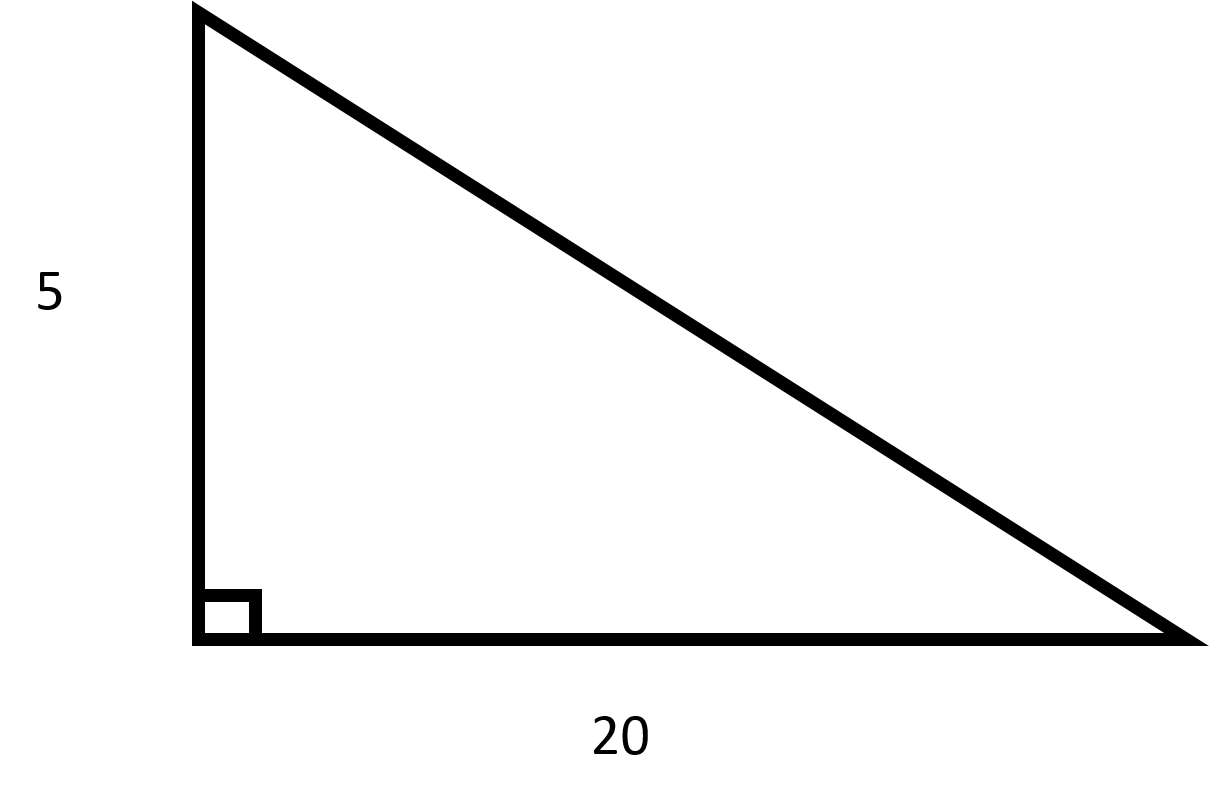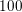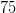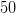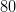Explanation:

Recall how to find the area of a triangle:Since this is a right triangle, the base and the height are the two leg lengths given.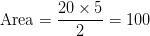### Example Question #50 : How To Find The Area Of A Right Triangle

Find the area.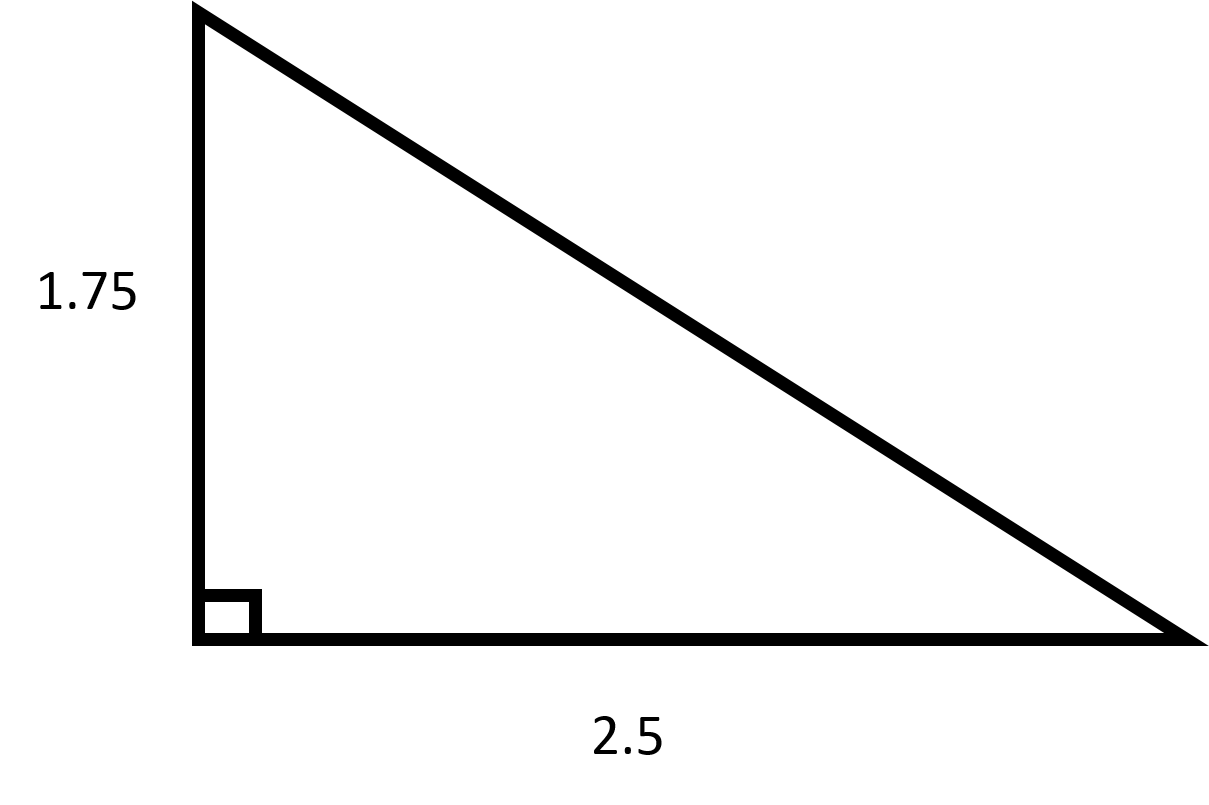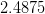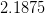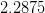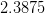Explanation:

Recall how to find the area of a triangle:Since this is a right triangle, the base and the height are the two leg lengths given.